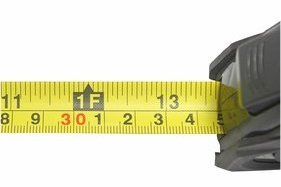## Length converterThis converter helps you to simultaneously convert a distance or a length from several common units like metre or meter (base unit of length in the International System of units - SI), foot, inch, kilometre, mile... Imperial units are a bit complicated : 1 mile = 1760 yards, 1 yard = 3 feet, 1 foot = 12 inches, 1 inch = 2.54 cm = 6 pica = 72 DTP points or pica points (unit used in typography)... So it is easier to use this converter ! Enter the length in the second column of the following array and the conversion will be performed automatically in all other units. The bottom of the array allows you to decompose your height in imperial English units ; ie, 175 cm = 5 foot 8 ¾ inches

Ångström Å
nanometre nm
micrometre (micron) µm
DTP point
pica point
millimetre mm
centimetre cm
inch in
foot ft
yard yd
metre m
kilometre km
mile mi
nautic mile nautic mile
Astronomic unit (Sun-Earth distance) au
ligth year ly
Parsec pc
 Decoding in imperial units : Mile Foot Inch 1/4 inch

The area of a square whose side length is as indicated in the table
The area of a circle whose radius length is as indicated in the table
The volume of a cube whose side length is as indicated in the table
The volume of a sphere whose length as radius is as indicated in the table

## Note

Lightyear is the distance light travels in one year.
One parsec is the distance at which one astronomical unit subtends an angle of one arcsecond. So 1 parsec=1/(tan(1'') au = 206 265 au = about 3.2616 lightyear.